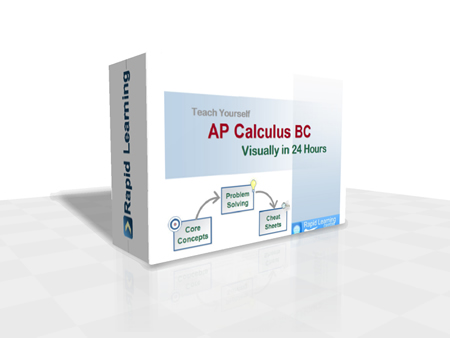How to Learn in 24 Hours?The Rapid Learning Movie

 Need Help? M-F: 9am-5pm (PST): Toll-Free: (877) RAPID-10 or 1-877-727-4310 24/7 Online Technical Support: The Rapid Support Center Secure Online Order:Need Proof? Testimonials by Our Users

 Rapid Learning Courses: MCAT in 24 Hours (2019-20) USMLE in 24 Hours (Boards) Chemistry in 24 Hours Biology in 24 Hours Physics in 24 Hours Mathematics in 24 Hours Psychology in 24 Hours SAT in 24 Hours ACT in 24 Hours AP in 24 Hours CLEP in 24 Hours DAT in 24 Hours (Dental) OAT in 24 Hours (Optometry) PCAT in 24 Hours (Pharmacy) Nursing Entrance Exams Certification in 24 Hours eBook - Survival Kits Audiobooks (MP3)

 Tell-A-Friend: Have friends taking science and math courses too? Tell them about our rapid learning system.Home »  Mathematics » High School AP Calculus BCCourse Preview

High School AP Calculus BC rapid learning series is designed specifically for high schoolers who are taking AP Calculus BC course or an advanced course with calculus I and II coverage. The rich-media Flash-based tutorials provide an much easier and non-intimidating way to master calculus, much like watching YouTube movies and playing interactive games. Learning calculus becomes easy and fun with narrated movies and problem drills, plus printable cheat sheets.

If you are visual and auditory learner and experiencing difficulty with traditional learning (lectures/textbooks), this rich-media course enables you to finally understand the concepts and problem solving via visual illustration and step-by-step problem solving, narrated by calculus educators.

1. 24x Core Concept Tutorials (Flash Movies)
Featuring concept map, illustrative concept, step-wise problem solving, real world application and summary with rich-media rendering and expert narration.
2. 24x Problem-Solving Drills (Flash Games)
Providing instant feedback on each answer choice, calculus tips to the problems, randomized problem sets, scoring system, time-tracking, play-by-play review and complete solutions at the end.
3. 24x Super Review Cheat Sheets (PDF Printables)
Offering at-a-glance review of all essential core concepts/formulas within a chapter, with one sheet per chapter, printable and laminatible Ideal for exam prep quick review.
Pre-requisite for Calculus: (1) High School Algebra 1- RL408 (2) High School Algebra 2 - RL401 (3) Trigonometry - RL402 (or Pre-Calculus - RL403).

High School AP Calculus BC Rapid Learning Series

This high school math course provides the topic by topic coverage required by College Board Adanced Placement Calculus BC. This is also an ideal course for any high school calculus for accelerated students.

Core Unit #1 – The Introduction

• Tutorial 01: Introduction to AP Calculus BC

Core Unit #2 – Limits and Continuity

• Tutorial 02: Limits and Continuity
• Tutorial 03: Rates of Change

Core Unit #3 – Derivatives

• Tutorial 04: The Derivative and the Tangent Line
• Tutorial 05: Differentiability and Rules of Differentiation
• Tutorial 06: The Chain Rule and Higher Derivatives
• Tutorial 07: Implicit Differentiation and Related Rates
• Tutorial 08: Applications of Differentiation - Part 1
• Tutorial 09: Applications of Differentiation - Part 2

Core Unit #4 – Integrals

• Tutorial 10: Riemann Sums and Definite Integrals
• Tutorial 11: The Fundamental Theorem of Calculus
• Tutorial 12: Antidifferentiation and Substitution Method
• Tutorial 13: Basic Integration Rules, Integration by Parts, and Partial Fraction
• Tutorial 14: Trigonometric Integrals and Trigonometric Substitution
• Tutorial 15: Numerical Integration
• Tutorial 16: Indeterminate Forms, L'Hopital's Rule and Improper Integrals
• Tutorial 17: Applications of Integration
• Tutorial 18: Differential Equations and Mathematical Modeling

Core Unit #5 – Special Topics

• Tutorial 19: Parametric Functions and Polar Functions
• Tutorial 20: Vector Functions
• Tutorial 21: Sequences, Series and Convergence
• Tutorial 22: Integral Test, Estimates of Sums and Comparison Test
• Tutorial 23: Alternating Series, the Ratio and Root Tests
• Tutorial 24: Power Series, Taylor and Maclaurin Series, Taylor Polynomials and Approximation

Master AP Calculus BC the rich-media way, get started now:

Course Preview